Electric Current Exam2 and Problem Solutions

Electric Current Exam2 and  Problem Solutions

1. Find the ratio of magnitude of two resistance made of same matter R1/R2?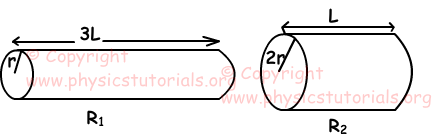R1=ρ.3L/(π.r2)

R2=ρ.L/(π.4r2)

R1/R2=12

2. Find the potential difference between points A and B.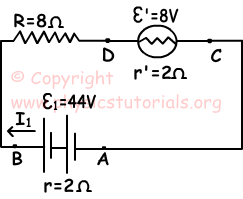Current passing through circuit is;

I=(ε-ε')/(R+r+r')=(44-8)/(8+2+2)

I=3 Amperes

Potential between points D and C;

VDC=-ε'-(Ir')=-8-(3.2)=-8-6=-14 Volt

Potential between points A and B;

VAB=ε-(I.r)=44-(3.2)

VAB=38 Volt

3. Find potential difference between points X and Y.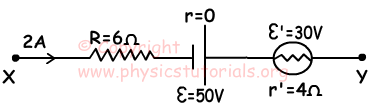VAB=VB-VA=Σε-ΣR.I

VAB=VB-VA=ε-ε'-(I.R+I.r')

VAB=50-30-2(6+4)

VAB=20-20=0

4. Find relation between heat produced by each branch of circuit given below.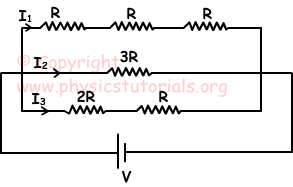Since branches are in parallel potential differences between them are equal.

I1=V/3R, I2=V/3R, I3=V/3R,

I1=I2=I3

W=I2.R.t

W1=W2=W3 and heat produced ;

Q1=Q2=Q3

5. If we close switches of circuits given below, find the changes in the brightness of the bulbs. (Neglect resistances of the batteries)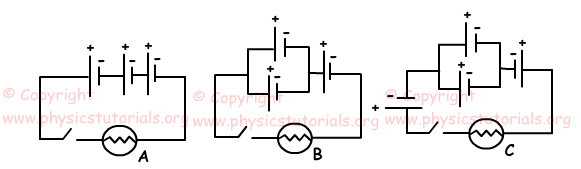When we close switches, currents on A, B and C are;
IA=3ε/R

IB=2ε/R

IC=ε/R

IA>IB>IC

Brightness of the bulbs is directly proportional to currents passing on them.

BA>BB>BC

Author: